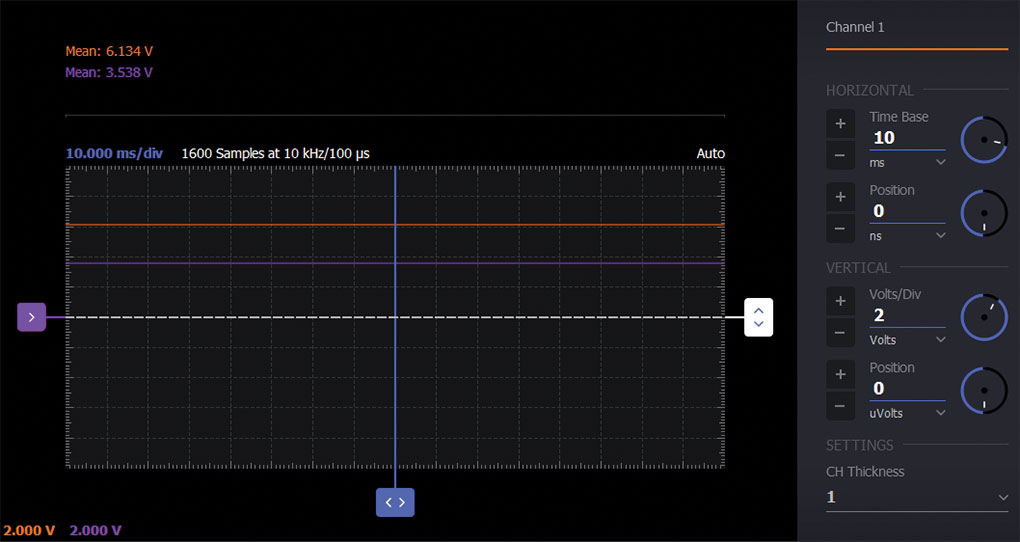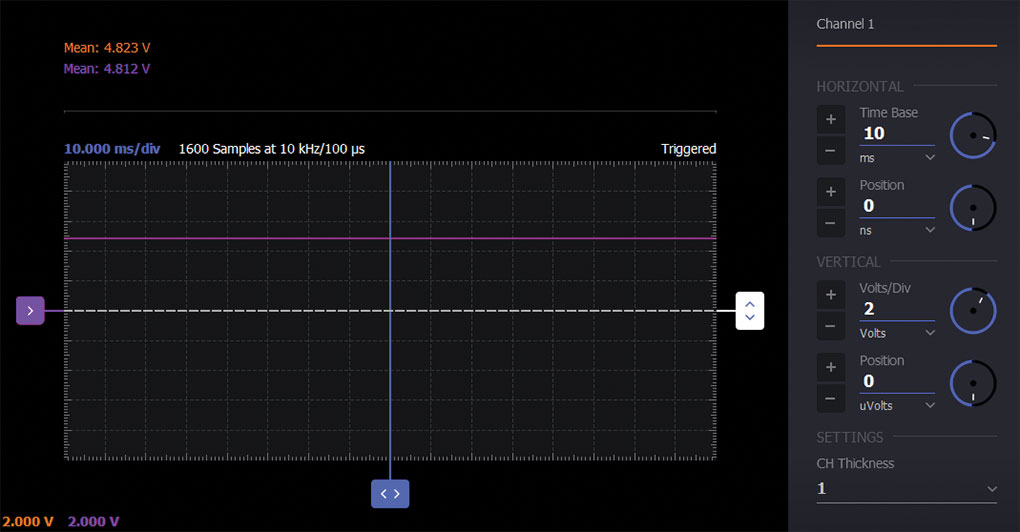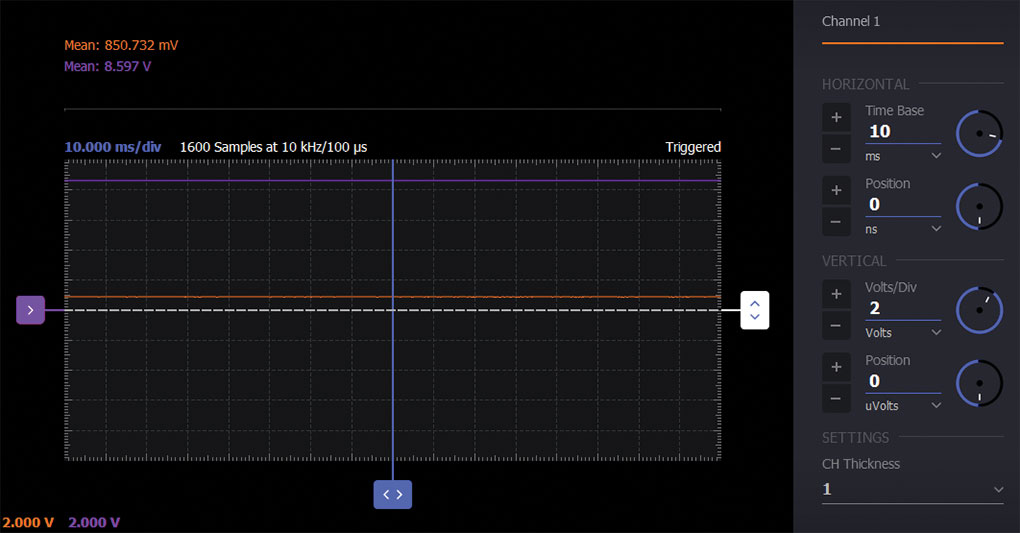### Objective:

A voltage regulator is a circuit used to maintain a constant output voltage at a load independent of changes in the load current. For example, the load could be a microcontroller-based system that requires a constant supply voltage even as its demand for current varies with system activity. The Zener diode regulator in Figure 1 offers a very simplified way to maintain the load voltage, VL, at the same value as the reverse breakdown voltage of the Zener diode, provided that the load resistance, RL, remains higher than some lower limit. The voltage source, VIN, and resistor, RS, model the Thévenin resistance of a possible circuit that has converted a high voltage such as the 120 V ac mains power to an unregulated and unfiltered lower dc voltage source.

### Materials:

• One 1 kΩ resistor (RS)
• One 5 kΩ variable resistor, potentiometer (RL)
• One Zener diode (1N4735 or similar)

### Directions:

Build the circuit shown in Figure 1 on your solderless breadboard using the 1N4735 6.2 V Zener diode. Use AWG1 (5 V constant) and the –5 V Vn user supply to establish the dc supply VIN. Use various fixed and variable resistors for RL.

### Procedure:

Step 1

Monitor and report the load voltage VL by using the Scopy Voltmeter instrument to measure VL for RL equal to:

• Open circuit (see Figure 3)
• 10 kΩ (see Figure 4)
• 1 kΩ (see Figure 5)
• 100 Ω (see Figure 6)Figure 3. RL = open circuit Zener diode regulator waveform.Figure 4. RL = 10 kΩ Zener diode regulator waveform.Figure 5. RL = 1 kΩ Zener diode regulator waveform.Figure 6. RL = 100 Ω Zener diode regulator waveform.

Step 2

Replace the load RL with a 5 kΩ potentiometer and adjust the potentiometer to determine the minimum value of RL for which VL remains within 10% of the Zener voltage, VZ. Measure and report the resistance to which you set the potentiometer. How does this resistance relate to the value of RS?

### Further Exploration

Investigate the current/voltage characteristic curve for the Zener diode using the same technique described in Step 2 by measuring the current in RS with Scope Channel 2 and plotting the voltage across the Zener vs. the current in the Oscilloscope XY mode. Be sure to adjust the horizontal voltage range and offset to include the 6.2 V breakdown voltage. Discuss your results, in particular the ways in which the Zener diode is similar to, and different from, a conventional diode.

As we saw in the simple Zener diode regulator in Figure 1, the maximum load current is determined by resistor RS. Also, the circuit is very inefficient for smaller load currents with respect to the maximum in that the excess current flows in the Zener when not flowing in the load. The inclusion of an emitter follower or Darlington emitter follower current amplifier can greatly improve the efficiency of this regulator circuit as shown in Figure 2.

• Two NPN transistors (2N3904 and TIP31)
• Two small signal diodes (1N914 or similar)

### Directions:

Build either of the circuits shown in Figure 2 on your solderless breadboard using the 1N4735 6.2 V Zener diode as D1 and a 2N3904 or TIP31 power transistor for Q1. Q2 can be a 2N3904 and D2, D3 can be a 1N914.

The extra diode D2 is added in series with the Zener to partially cancel the additional VBE drop due to the emitter follower Q1. Likewise, in the Darlington configuration, two diodes (D2, D3) are added to again partially cancel the two VBE drop of the Darlington follower.

### Question:

Using the circuit in Figure 1, compute the resistance, RL, for which the VL value is at 20% of the Zener voltage VZ.

You can find the answers at the StudentZone blog.